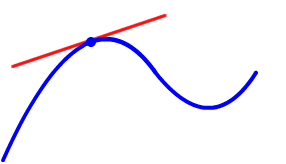Here's a Calculus preview of WHY slopes of lines and equations of lines will be so important.

Tangent lines!

So, what's a tangent line?

First of all, don't think of the tangent from trig...  Yeah, it's definitely related, but we don't have to think that hard here.

Check out this picture:A tangent line is a line that touches a graph in one local point so that, when you zoom in on it, the graph and the tangent line will eventually look the same.Why will we need this in Calculus?

Because we want to study the exact behavior of graphs at every little point and section...  Lines are much easier to work with than the entire graph.  Tangent lines will give us easy snapshots of what's going on!